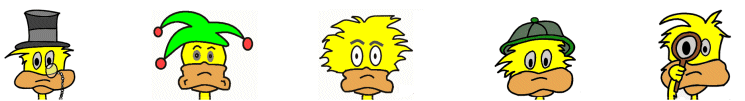# Kids Math

## Glossary and Terms: Graphs and Lines

Abscissa - The horizontal line, or x-axis, of a graph.

Arc - A portion of the circumference of a circle.

Axis - One of the lines that is used to form a graph. There is the horizontal x-axis and the vertical y-axis in a two dimensional graph.Example of x-axis, y-axis, and coordinates on a graph

Bisect - To bisect an object is to divide it into two equal halves.

Collinear - A set of three or more points that are lying on the same straight line are collinear.

Coordinates - A set of two numbers that indicate where a point is on a graph. The first number indicates the x-axis and the second number the y-axis. Other names include ordered pair and numbered pair.

Coplanar lines - Two or more lines that are on the same plane or flat surface.

Diameter - A line segment that passes through the center of a circle with each end point being on the circumference.

Endpoint - The point at the end of a line segment or ray.

Horizontal - A flat or level line or plane that is perpendicular to the vertical.

Intersecting lines - Two or more lines that meet at a point are intersecting.

Line - A straight object that is infinitely long and thin. It is only in one dimension.

Line segment - A portion of a line with two endpoints.

Midpoint - The point of a line segment that is the same distance from both endpoints.

Noncollinear points - A set of three points that are not located on the same line.

Number pair - Two numbers that represent a point on a graph, also called the coordinates.

Ordinate - The vertical line, or y-axis, of a graph.

Origin - The origin is the point where the X and Y axis intersect on a graph. This is the point (0,0) in a two-dimensional graph.

Parallel lines - Lines that never intersect or cross are parallel lines.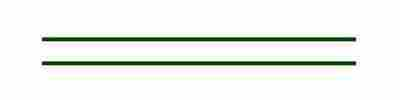Parallel lines

Perpendicular lines - Two lines that form a right angle (90 degrees) are perpendicular lines.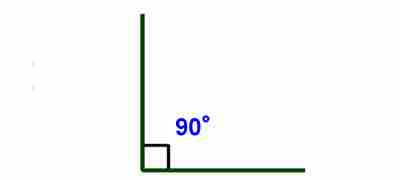Perpendicular lines

Ray - A line that has one endpoint, but extends forever in one direction.

Slope - A number that indicates the incline or steepness of a line on a graph. Slope equals the "rise" over the "run" of a line on a graph. This can also be written as the change in y over the change in x.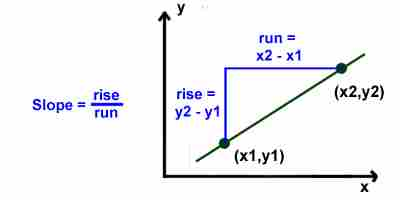Example: If two points on a line are (x1, y1) and (x2, y2), then the slope = (y2 - y1) ÷ (x2-x1).

Tangent - A line that touches an object such as an arc or circle at a single point.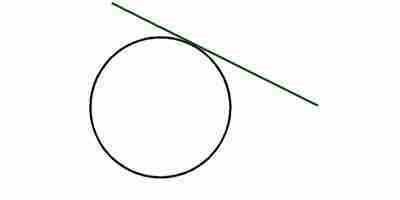The green line is tangent to the circle

Transversal - A transversal is a line that crosses two or more other lines.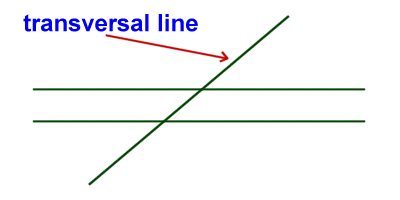Vertical - A line or plane that is upright and perpendicular to the horizontal.

More Math Glossaries and Terms

Algebra glossary
Angles glossary
Figures and Shapes glossary
Fractions glossary
Graphs and lines glossary
Measurements glossary
Mathematical operations glossary
Probability and statistics glossary
Types of numbers glossary
Units of measurements glossary

Back to Kids Math

Back to Kids Study